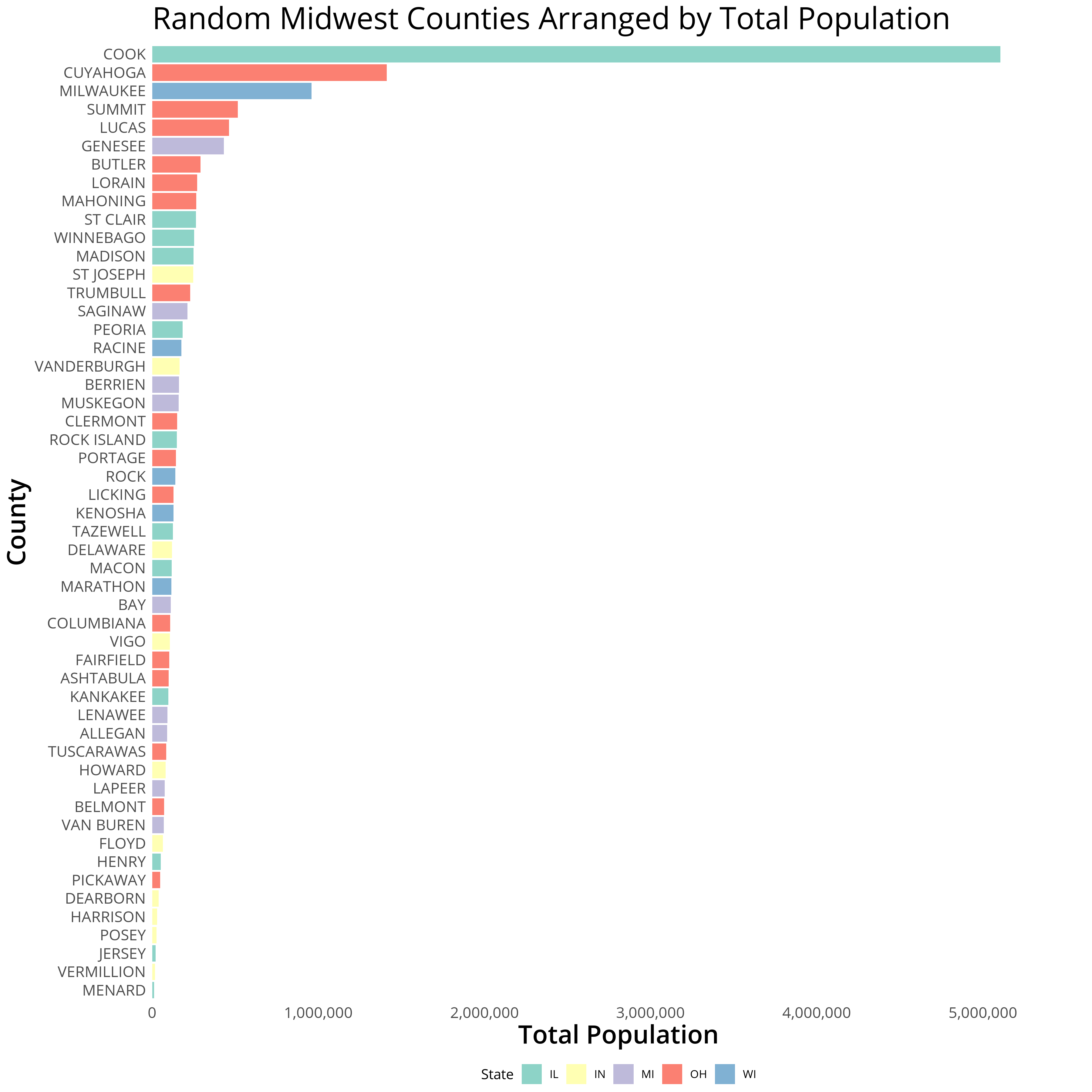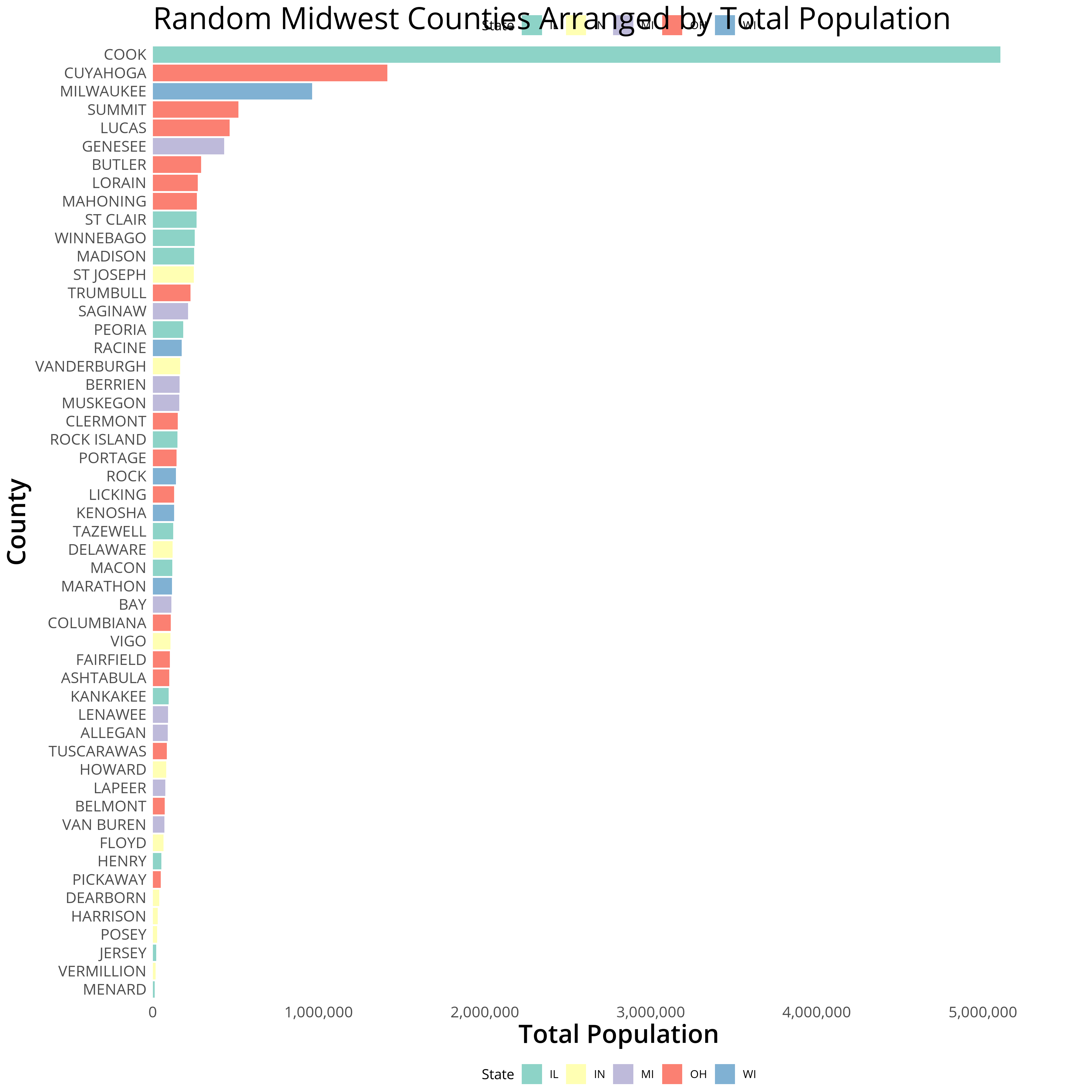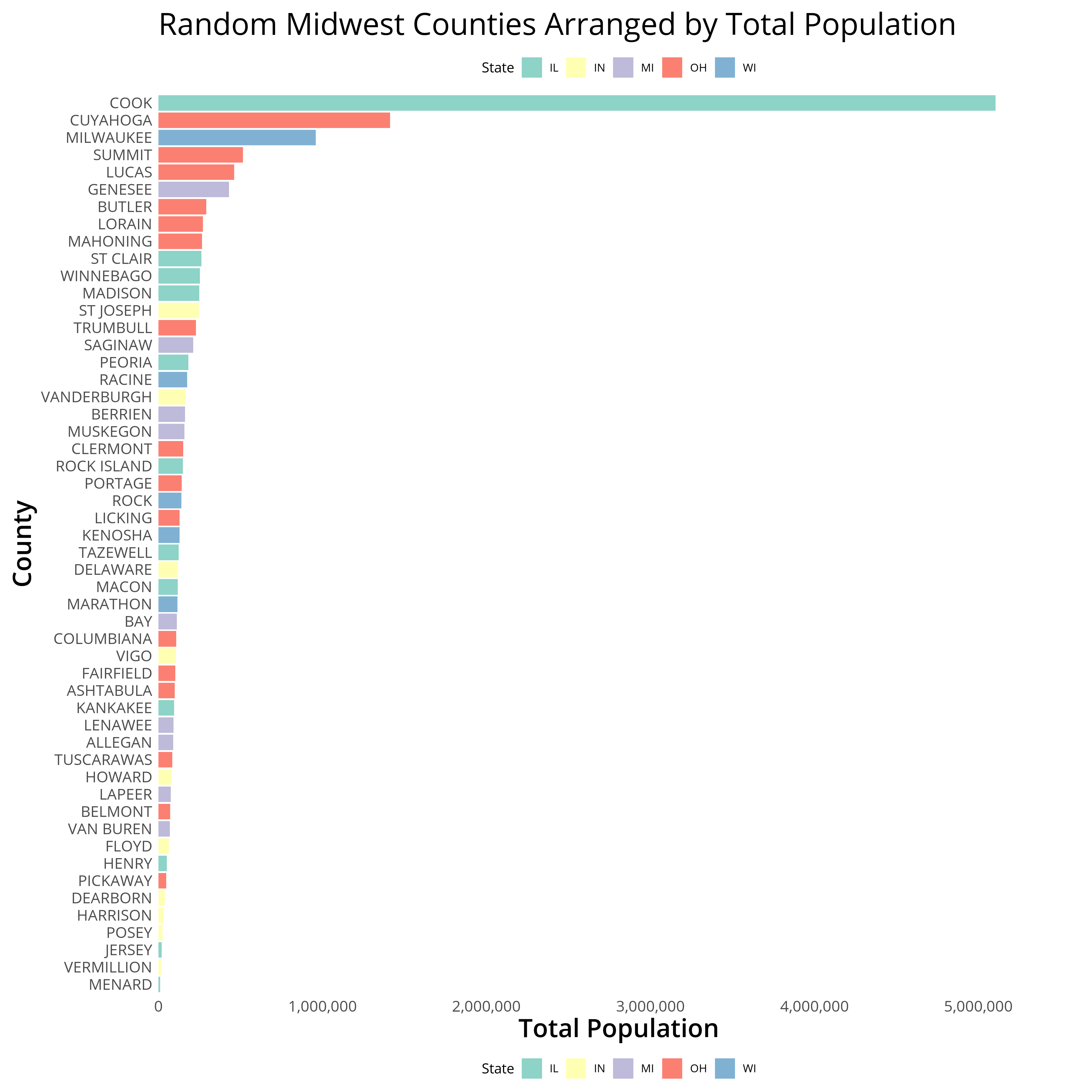## Mastering the R package `ggplot2`

The `ggplot2` package provides powerful methods to display data as graphics. The beauty of the package lies in it’s simplicity - understanding the core methods (applying variables to aesthetics and transformations) covers ~95% of static visualizations a data visualization developer might be interested in generating. Most of final 5% can be achieved by understanding the infrastructure of the package. One such example is how plot components are “written” to the graphics device.

I’ll walk through how legends are generated and how we can create a second duplicate legend to bookend the top and bottom of a long bar plot. I I contributed a method to solve this problem on stackoverflow and wanted to get into some further details in this post.

Let’s walk through creating a second legend in ggplot2.

## Creating Dual Legends in ggplot2 - Libraries and Data Reshaping

The `midwest` data set from the `ggplot2` package contains demographic information of midwest counties and should work as a representative dummy data set for this post. Here is a quick overview of the `midwest` data set using `glimpse`:

R Libraries, `Midwest` data set overview
``````    # load libraries and fonts
library(ggplot2)
library(scales)
library(dplyr)
library(grid)
library(extrafont)
glimpse(midwest)``````

I’ll subset the data a little bit to accomodate the plot and organize the display order of counties by their total population. The `theme_white` block of code adds some basic aesthetics and fonts.

R Libraries, Data Manipulation, and Plot Generation
``````    # load libraries and fonts
library(ggplot2)
library(scales)
library(grid)
library(extrafont)

# data subset and refactoring
midwest <- midwest[!duplicated(midwest\$county), ]
midwestAAU <- midwest %>% filter(category == "AAU") %>% arrange(poptotal)
midwestAAU\$county <- factor(midwestAAU\$county,levels = midwestAAU\$county,labels = toupper(midwestAAU\$county))

gg <- ggplot(midwestAAU) + geom_bar(aes(y = poptotal, x = county, fill = state), stat = "identity") +
scale_y_continuous(expand = expand_scale(mult = c(0, .1)), labels = comma) + coord_flip() + theme_minimal() + labs(title = "Random Midwest Counties Arranged by Total Population",y = "Total Population", x  = "County", fill = "State") + scale_fill_brewer(palette = "Set3")

# plot aesthetics
theme_white <- theme(text = element_text(family="Open Sans"),
panel.grid.major.y=element_blank(),
panel.grid.major.x=element_blank(),
panel.grid.minor.x=element_blank(),
panel.grid.minor.y=element_blank(),
plot.title=element_text(size=24,family = "Open Sans",lineheight=.75),
axis.title.x=element_text(size=20, family = "Open Sans Semibold"),
axis.title.y=element_text(size=20,family = "Open Sans Semibold"),
axis.text.x=element_text(size=12),
axis.text.y=element_text(size=12),
axis.ticks = element_blank(),
legend.position = "bottom",
legend.margin = margin(b = 0)
)

# apply theme and export plot
gg <- gg + theme_white
ggsave(gg, filename = "midwestPlot.png",height = 12, width = 12, dpi = 300, units = "in", device='png')``````Now that we’ve generated our plot we can focus on creating the second legend.

## Creating a Second Legend in `ggplot2`

So far we’ve covered `ggplot2` functionalities that should create the ~95% of plots I discussed earlier. To expand upon these, let’s get into some `ggplot2` internals. The function `ggplotGrob` allows us to parse our saved `gg` graphical object. This object can be manipulated to override default `ggplot2` conventions or provide methods to hack our plot in ways that the package isn’t designed for intentionally (i.e. where there isn’t a compiled function.)

The `createTopLegend` function below easily duplicates a bottom legend at the top of the plot by:

1. Grabbing the ggplot graphical object
2. Retrieving the legendGrob within the ggplot object
3. Duplicating the legendGrob layout
4. Specifying the location of the new legendGrob
5. Appending the new legendGrob to the ggplot object
createTopLegend Function
``````    createTopLegend <- function(ggplot, heightFromTop = 1) {
# grab the saved ggplot2 object
g <- ggplotGrob(ggplot)

# count the number of grobs in this plot (which we'll use to append another)
nGrobs <- (length(g\$grobs))

# find the guide-box object which provides the plot information for the legend
legendGrob <- which(g\$layout\$name == "guide-box")

# duplicate the legend's grob and layout
g\$grobs[[nGrobs+ 1]] <- g\$grobs[[legendGrob]]
g\$layout[nGrobs+ 1,] <- g\$layout[legendGrob,]

# g\$layout\$t <- ifelse( g\$layout\$t > heightFromTop, g\$layout\$t + 1, g\$layout\$t)

# retrieve the alignment of the legend
rightLeft <- unname(unlist(g\$layout[legendGrob, c(2,4)]))

# specify the location of the new legendGrob (t,r,b,l)
# use the heightFromTop argument to adjust the vertical positioning
g\$layout[nGrobs+ 1, 1:4] <- c(heightFromTop, rightLeft, heightFromTop, rightLeft)
g
}``````

We can then apply the `createTopLegend` function on our saved `ggplot2` object `gg` and redraw our plot with `grid.draw`:

Plot with Duplicate Legend…Overlapping
``````    gg2 <- createTopLegend(gg, 3)
ggsave(grid.draw(gg2), filename = "midwestPlot2.png",height = 12, width = 12, dpi = 300, units = "in", device='png')``````You’ll notice that our top legend now overlaps with the positioning of the title. To remedy this we can add some additional margins from within `theme_white`. We’ll add a bottom margin to the title to add spacing, a bottom margin to the legends, and a negative margin to the bottom of the plot. Each of these margins work in tandem so the negative plot margin is necessary to account for the extra spacing we’re adding to the top legend for the plot to be appropriately spaced.

Fiddling with Title, Legend, and Plot Margins to Accommodate for the Top Legend
``````    # plot aesthetics
theme_white <- theme_white + theme(
plot.title=element_text(size=24,family = "Open Sans",lineheight=.75, margin = margin(b = 40)),
legend.margin = margin(b = 40),
plot.margin = margin(t = 10, r = 10, b = -30, l = 10)

)
gg <- gg + theme_white

gg2 <- createTopLegend(gg, heightFromTop = 4)
ggsave(grid.draw(gg2), filename = "midwestPlotLegend.png",height = 12, width = 12, dpi = 300, units = "in", device='png')``````Interested in learning more? Hire me to consult on your next project, follow me on twitter, or contact me via email. All inquiries welcome!